# The missing method: Deleting from Okasaki's red-black trees

Balanced-tree-based maps are a workhorse in functional programming.

Because of their disarming simplicity, Chris Okasaki's purely functional red-black trees are a popular means for implementing such maps.

In his book, self-balancing binary search trees are less than a page of code.

Except that delete is left as an exercise to the reader.

Unfortunately, deletion is tricky.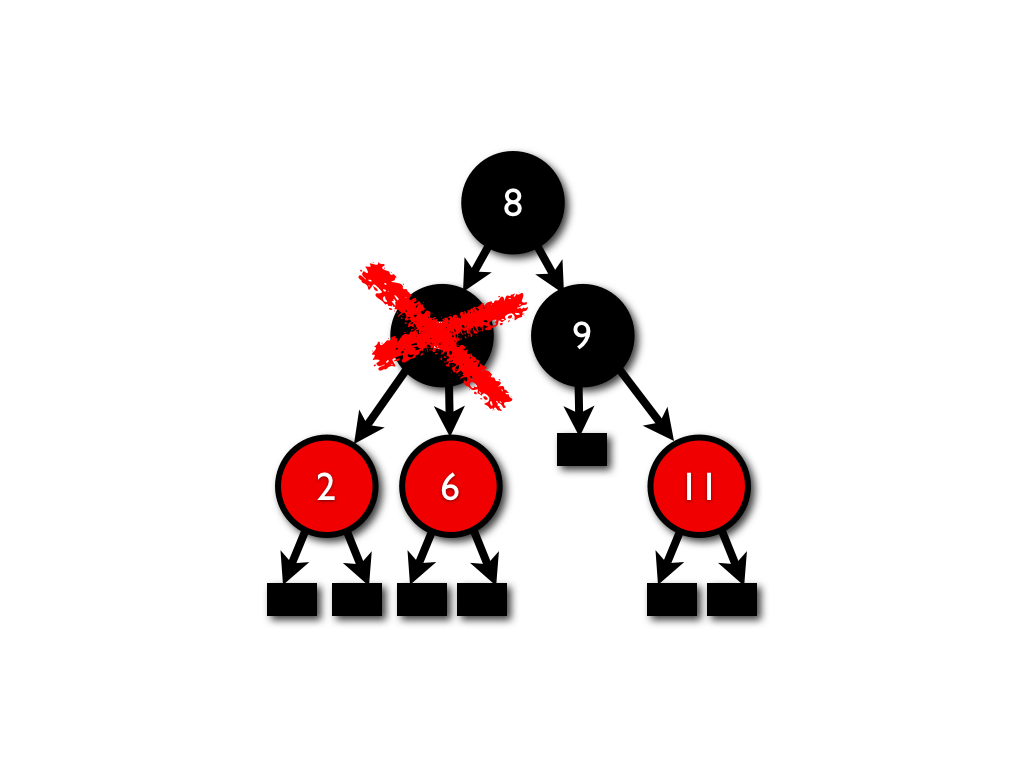Stefan Kahrs devised a widely-copied functional red-black delete.

But, the Kahrs algorithm is complex, because Stefan's primary goal was to enhance correctness by enforcing the red-black invariants with types.

I wondered whether a simple, efficient, purely functional, "obviously correct" red-black delete algorithm is possible.

Of course, it is.

By temporarily allowing two new colors during the deletion process--double-black and negative black--it's easy to factor delete into three conceptually simple phases: remove, bubble and balance.

Read on for the details of my implementation in Racket.

Update: Wei Hu emailed me to point out that two of my cases for `remove` are unnecessary, and can be deleted. The algorithm is even simpler now!

Update: An algorithm inspired by this post has been published in the Journal of Functional Programming: Deletion: The curse of the red-black tree.

Update on 18 Aug 2014: Stephanie Weirich produced a GADT version in Haskell, and I have a separate article on using QuickCheck to debug my own Haskell code.

## Red-black trees

Red-black trees are self-balancing binary search trees in which every node has one of two colors: red or black.

Red-black trees obey two additional invariants:

1. Any path from the root to a leaf has the same number of black nodes.
2. All red nodes have two black children.

Leaf nodes, which do not carry values, are considered black for the purposes of both height and coloring.

Any tree that obeys these conditions ensures that the longest root-to-leaf path is no more than double the shortest root-to-leaf path. These constraints on path length guarantee fast, logarithmic reads, insertions and deletes.

### Examples

The following is a valid red-black tree: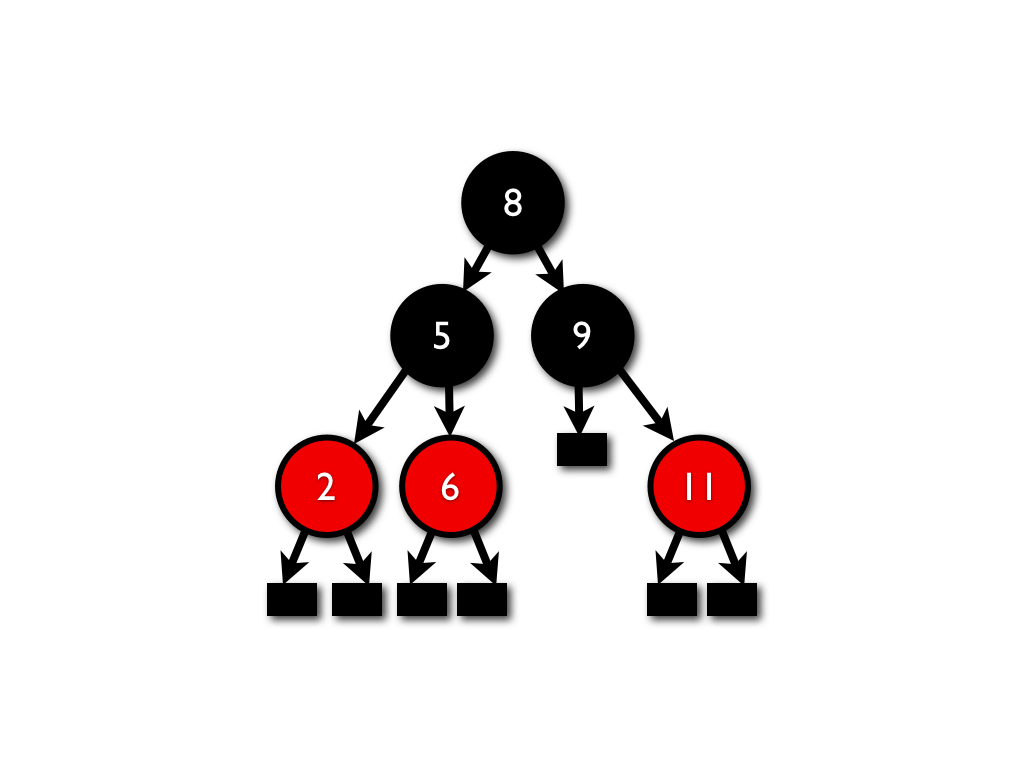Both of the following are invalid red-black representations of the set {1,2,3}:The following are valid representations of the set {1,2,3}: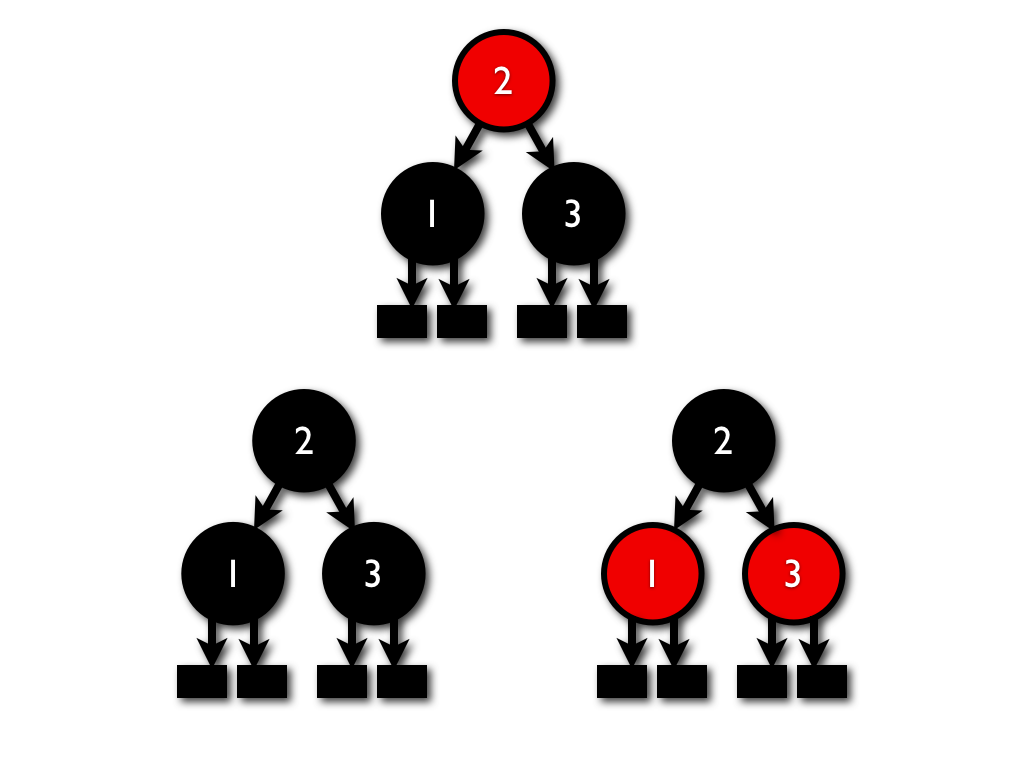## Delete: A high-level summary

There are many easy cases in red-black deletion--cases where the change is local and doesn't require rebalancing or (much) recoloring.

The only hard case ends up being the removal of a black node with no children, since it alters the height of the tree.

Fortunately, it's easy to break apart this case into three phases, each of which is conceptually simple and straightforward to implement.

The trick is to add two temporary colors: double black and negative black.

The three phases are then removing, bubbling and balancing:

1. By adding the color double-black, the hard case reduces to changing the target node into a double-black leaf. A double-black node counts twice for black height, which allows the black-height invariant to be preserved.
2. Bubbling tries to eliminate the double black just created by a removal. Sometimes, it's possible to eliminate a double-black by recoloring its parent and its sibling. If that's not possible, then the double-black gets "bubbled up" to its parent. To do so, it might be necessary to recolor the double black's (red) sibling to negative black.
3. Balancing eliminates double blacks and negative blacks at the same time. Okasaki's red-black algorithms use a rebalancing procedure. It's possible to generalize this rebalancing procedure with two new cases so that it can reliably eliminate double blacks and negative blacks.

## Red-black trees in Racket

My implementation of red-black trees is actually an implementation of red-black maps:

```; Struct definition for sorted-map:
(define-struct sorted-map (compare))

;  Internal nodes:
(define-struct (T sorted-map)
(color left key value right))

;  Leaf nodes:
(define-struct (L sorted-map) ())

;  Double-black leaf nodes:
(define-struct (BBL sorted-map) ())
```

Every `sorted-map` has a comparison function on keys. Each internal node (`T`) has a color, a left sub-tree, a key, a value and a right sub-tree. There are also black leaf nodes (`L`) and double-black leaf nodes (`LBB`).

The implementation contains four colors total--double black (`'BB`), black (`'B`), red (`'R`) and negative black (`'-B`):To make the expression of routines and sub-routines compact and readable, I used Racket's fully extensible pattern-matching systems:

```; Matches internal nodes:
(define-match-expander T!
(syntax-rules ()
[(_)            (T _ _ _ _ _ _)]
[(_ l r)        (T _ _ l _ _ r)]
[(_ c l r)      (T _ c l _ _ r)]
[(_ l k v r)    (T _ _ l k v r)]
[(_ c l k v r)  (T _ c l k v r)]))

; Matches leaf nodes:
(define-match-expander L!
(syntax-rules ()
[(_)     (L _)]))

; Matches black nodes (leaf or internal):
(define-match-expander B
(syntax-rules ()
[(_)              (or (T _ 'B _ _ _ _)
(L _))]
[(_ cmp)          (or (T cmp 'B _ _ _ _)
(L cmp))]
[(_ l r)          (T _ 'B l _ _ r)]
[(_ l k v r)      (T _ 'B l k v r)]
[(_ cmp l k v r)  (T cmp 'B l k v r)]))

; Matches red nodes:
(define-match-expander R
(syntax-rules ()
[(_)              (T _ 'R _ _ _ _)]
[(_ cmp)          (T cmp 'R _ _ _ _)]
[(_ l r)          (T _ 'R l _ _ r)]
[(_ l k v r)      (T _ 'R l k v r)]
[(_ cmp l k v r)  (T cmp 'R l k v r)]))

; Matches negative black nodes:
(define-match-expander -B
(syntax-rules ()
[(_)                (T _ '-B _ _ _ _)]
[(_ cmp)            (T cmp '-B _ _ _ _)]
[(_ l k v r)        (T _ '-B l k v r)]
[(_ cmp l k v r)    (T cmp '-B l k v r)]))

; Matches double-black nodes (leaf or internal):
(define-match-expander BB
(syntax-rules ()
[(_)              (or (T _ 'BB _ _ _ _)
(BBL _))]
[(_ cmp)          (or (T cmp 'BB _ _ _ _)
(BBL _))]
[(_ l k v r)      (T _ 'BB l k v r)]
[(_ cmp l k v r)  (T cmp 'BB l k v r)]))
```

To further condense cases, the implementation also uses color arithmetic. For instance, adding a black to a black yields a double-black. Subtracting a black from a black yields a red. Subtracting a black from a red yields a negative black. In Racket:

```(define/match (black+1 color-or-node)
[(T cmp c l k v r)  (T cmp (black+1 c) l k v r)]
[(L cmp)            (BBL cmp)]
['-B 'R]
['R  'B]
['B  'BB])

(define/match (black-1 color-or-node)
[(T cmp c l k v r)  (T cmp (black-1 c) l k v r)]
[(BBL cmp)          (L cmp)]
['R   '-B]
['B    'R]
['BB   'B])
```

Diagrammatically: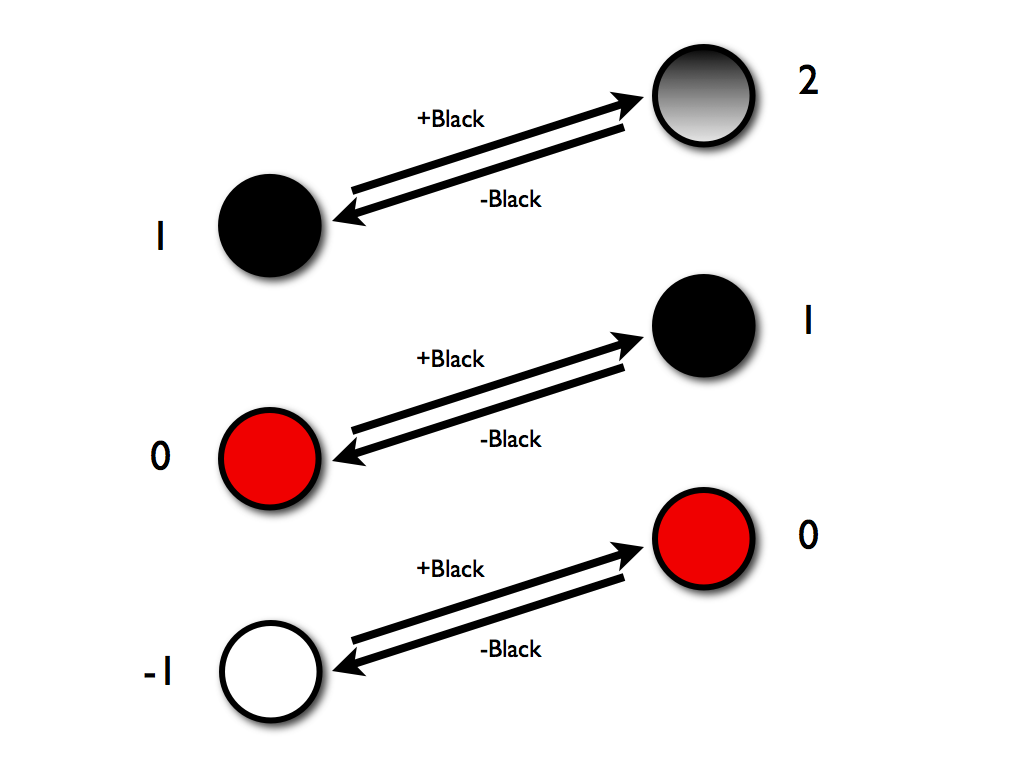## Red-black deletion in detail

In Racket, the skeleton for red-black deletion is:

```(define (sorted-map-delete node key)

; The comparison function on keys:
(define cmp (sorted-map-compare node))

; Finds and deletes the node with the right key:
(define (del node) ...)

; Removes this node; it might
; leave behind a double-black node:
(define (remove node) ...)

; Kills a double-black, or moves it upward;
; it might leave behind a negative black:
(define (bubble c l k v r) ...)

; Removes the max (rightmost) node in a tree;
; may leave behind a double-black at the root:
(define (remove-max node) ...)

; Delete the key, and color the new root black:
(blacken (del node)))
```

### Finding the target key

The procedure `del` searches through the tree until it finds the node to delete, and then it calls `remove`:

```  (define/match (del node)
[(T! c l k v r)
; =>
(switch-compare (cmp key k)
[<   (bubble c (del l) k v r)]
[=   (remove node)]
[>   (bubble c l k v (del r))])]

[else     node])
```

(`define/match` and `switch-compare` are macros to make the code more compact and readable.)

Because deletion could produce a double-black node, the procedure `bubble` gets invoked to move it upward.

### Removal

The `remove` procedure breaks removal into several cases:

The cases group according to how many children the target node has. If the target node has two sub-trees, `remove` reduces it to the case where there is at most one sub-tree.

It's easy to turn removal of a node with two children into removal of a node with at most one child: find the maximum (rightmost) element in its left (less-than) sub-tree; remove that node instead, and place its value into the node to be removed.

For example, removing the blue node (with two children) reduces to removing the green node (with one) and then overwriting the blue with the green: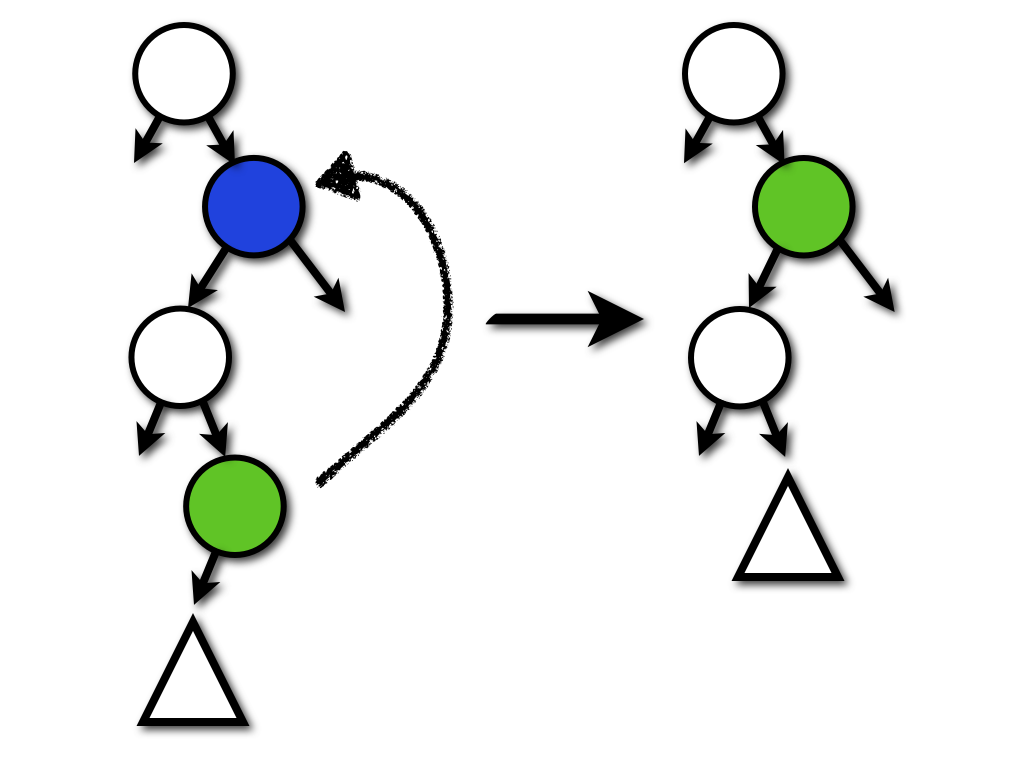If the target node has leaves for children, removal is straightforward:A red node becomes a leaf node; a black node becomes a double-black leaf.

If the target node has one child, there is only one possible case. (I originally thought there were three, but Wei Hu pointed out that the other two violate red-black constraints, and cannot happen.)

That single case is where the target node is black and its child is red.

The child becomes the parent, and it is made black:The corresponding Racket code for these cases is:

```  (define/match (remove node)
; Leaves are easy to kill:
[(R (L!) (L!))     (L cmp)]
[(B (L!) (L!))     (BBL cmp)]

; Killing a node with one child:
[(or (B (R l k v r) (L!))
(B (L!) (R l k v r)))
; =>
(T cmp 'B l k v r)]

; Killing a node with two sub-trees:
[(T! c (and l (T!)) (and r (T!)))
; =>
(match-let (((cons k v) (sorted-map-max l))
(l*         (remove-max l)))
(bubble c l* k v r))])
```

For the record, these were the two cases that got eliminated:### Bubbling

The `bubble` procedure moves double-blacks from children to parents, or eliminates them entirely if possible.

There are six possible cases in which a double-black child appears:In every case, the action necessary to move the double black upward is the same; a black is substracted from the children, and added to the parent: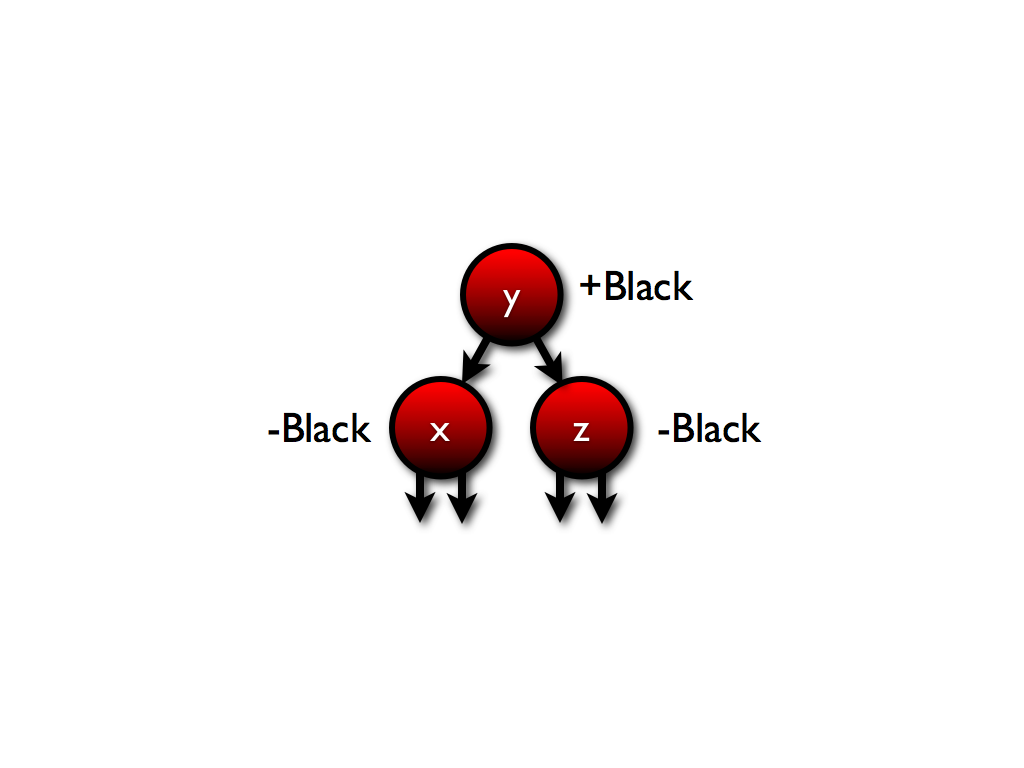This operation leads to the corresponding trees: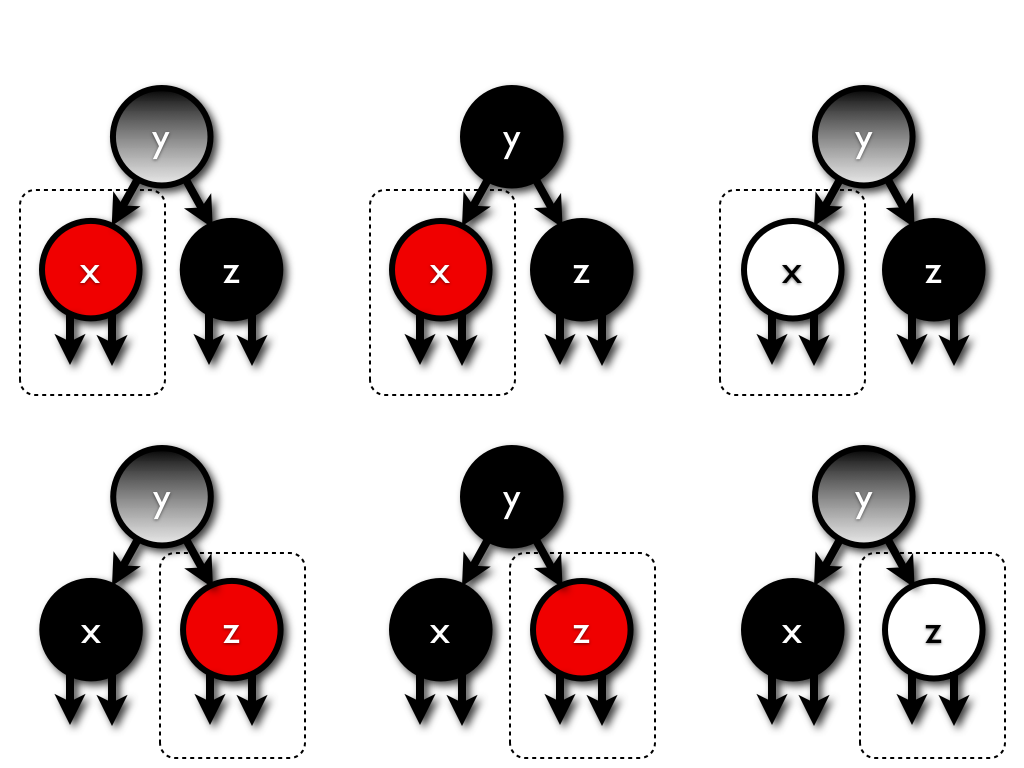A dotted line indicates the need for a rebalancing operation, because of the possible introduction of a red/red or negative black/red parent/child relationship.

Because the action is the same in every case, the code for bubble is short:

```  (define (bubble c l k v r)
(cond
[(or (double-black? l) (double-black? r))
; =>
(balance cmp (black+1 c) (black-1 l) k v (black-1 r))]

[else (T cmp c l k v r)]))
```

### Generalizing rebalancing

Okasaki's balancing operation takes a tree with balanced black-height but improper coloring and performs a tree rotation and a recoloring.

The original procedure focused on fixing red/red violations. The new procedure has to fix negative-black/red violations, and it also has to opportunistically eliminate double-blacks.

The original procedure eliminated all of the red/red violations in these trees:by turning them into this tree: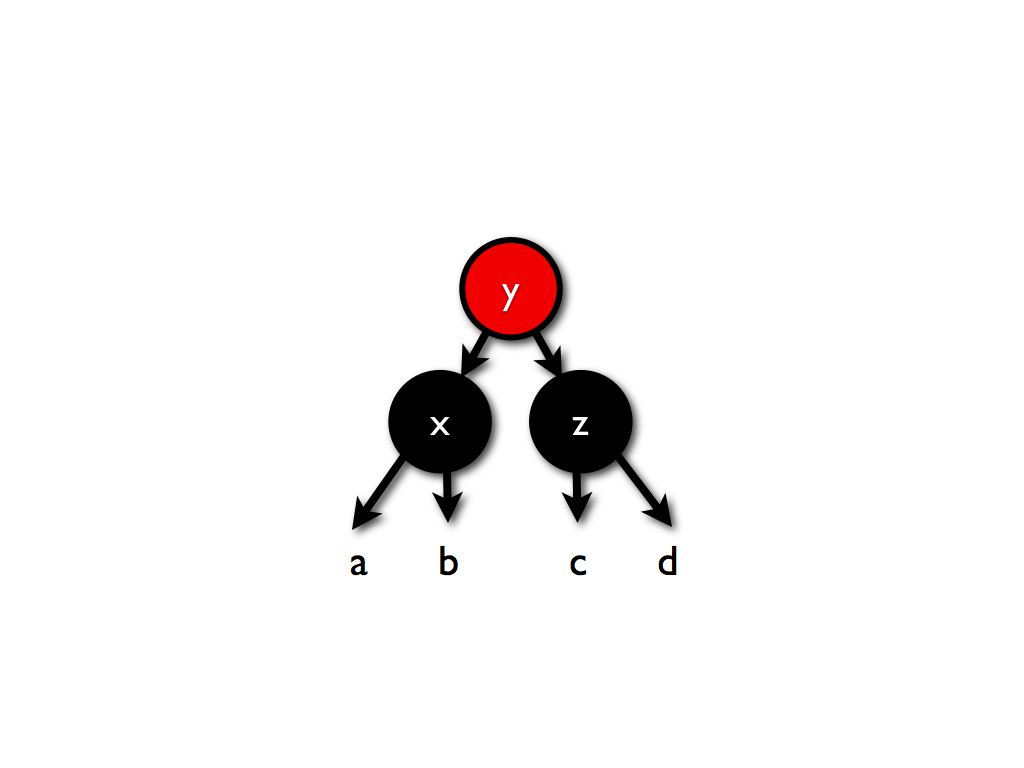The extended procedure can handle a root that is double-black: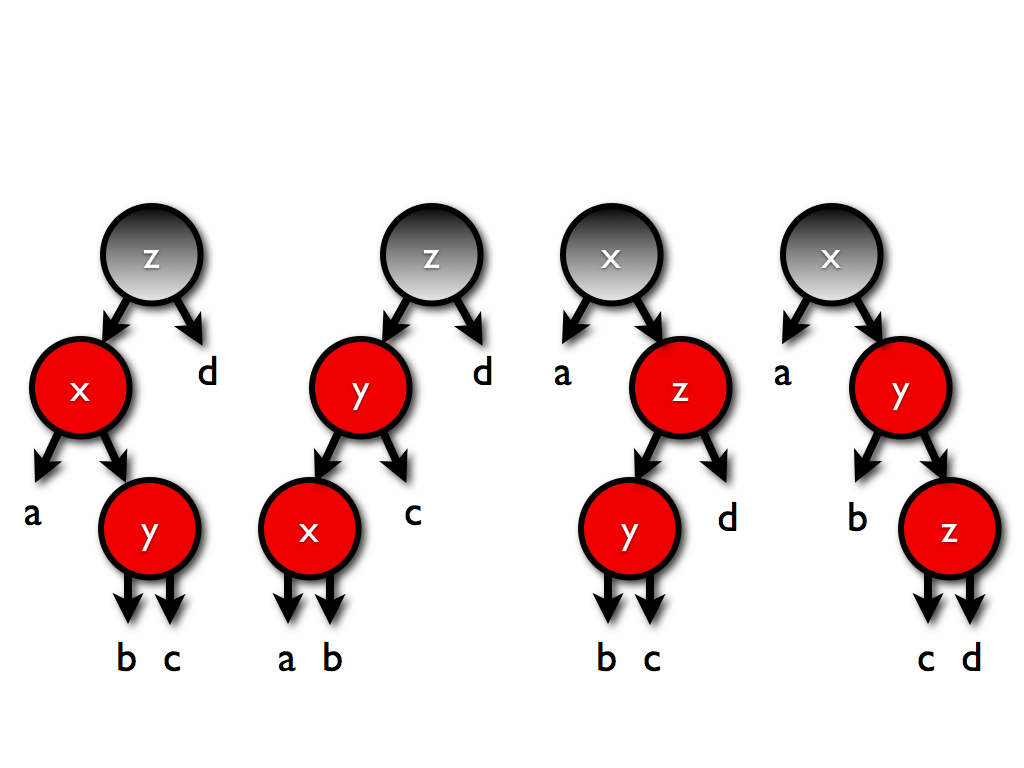by turning them all into this tree:If a negative black appears as the result of a bubbling, as in: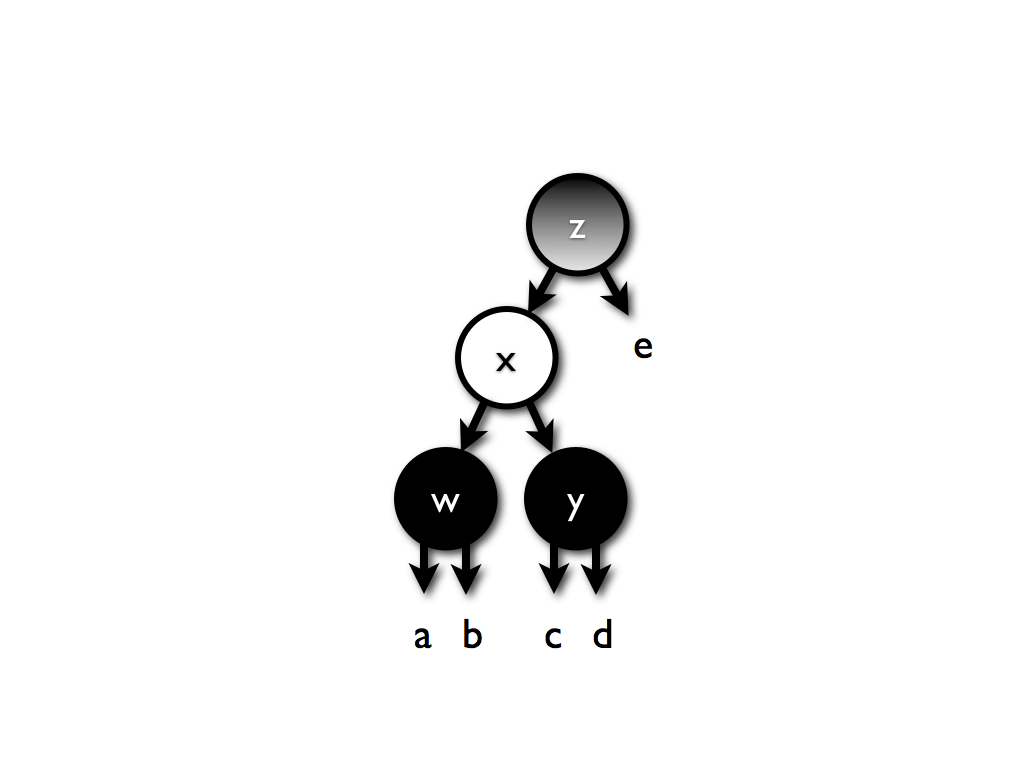then a slightly deeper transformation is necessary:Once again, the dotted lines indicate the possible introduction of a red/red violation that could need rebalancing.

So, the balance procedure is recursive, but it won't call itself more than once.

There is also the symmetric case for this last operation, and these two new cases take care of all possible negative blacks.

In Racket, only two new cases are added to the balancing procedure:

```; Turns a black-balanced tree with invalid colors
; into a black-balanced tree with valid colors:
(define (balance-node node)
(define cmp (sorted-map-compare node))
(match node

; Classic balance, but also catches double blacks:
[(or (T! (or 'B 'BB) (R (R a xk xv b) yk yv c) zk zv d)
(T! (or 'B 'BB) (R a xk xv (R b yk yv c)) zk zv d)
(T! (or 'B 'BB) a xk xv (R (R b yk yv c) zk zv d))
(T! (or 'B 'BB) a xk xv (R b yk yv (R c zk zv d))))
; =>
(T cmp (black-1 (T-color node))
(T cmp 'B a xk xv b)
yk yv
(T cmp 'B c zk zv d))]

; Two new cases to eliminate negative blacks:
[(BB a xk xv (-B (B b yk yv c) zk zv (and d (B))))
; =>
(T cmp 'B (T cmp 'B a xk xv b)
yk yv
(balance cmp 'B c zk zv (redden d)))]

[(BB (-B (and a (B)) xk xv (B b yk yv c)) zk zv d)
; =>
(T cmp 'B (balance cmp 'B (redden a) xk xv b)
yk yv
(T cmp 'B c zk zv d))]

[else     node]))

(define (balance cmp c l k v r)
(balance-node (T cmp c l k v r)))
```
And, that's it.

## Code

The code is available as a Racket module. My testing script is also available:

The testing system uses a mixture of exhaustive testing (on all trees with up to eight elements) and randomized testing (on much larger trees).

I'm confident it flushed the bugs out of my implementation. Please let me know if you find a test case that breaks it.

Update: By popular demand, I created an implementation of this algorithm for red-black-tree-backed sets in Haskell: RedBlackSet.hs.

I wrote a separate blog post on using QuickCheck to debug my Haskell translation.

Stephanie Weirich created a GADT version of the algorithm.

Burke Fetscher created a PLT Redex model for red-black trees that can be used for randomized testing.

### More resources

• Every functional programmer should have a copy of Chris Okasaki's Purely Functional Data Structures.
• Richard Bird's brand new Pearls of Functional Algorithm Designis a fantastic case-study book on the design of elegant functional algorithms.